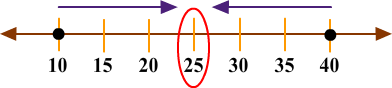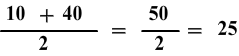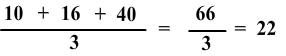# Mean

Print Rate 0 stars
Lesson size:
Message preview:
Someone you know has shared lesson with you:

To play this lesson, click on the link below:

https://www.turtlediary.com/lesson/mean.html

Hope you have a good experience with this site and recommend to your friends too.

Login to rate activities and track progress.
Login to rate activities and track progress.

The mean is the average of a set of numbers.

It is calculated by dividing the sum of all the items in a set by the total number of items in the set.

## Set of Numbers

A group of numbers can be referred to as a set.

Example

a.)15, 4, 37, 18, 11

b.) 6, 6, 7, 9, 11, 11, 15, 20

## Mean of two numbers

When there are only two numbers, the mean is easy to find. It is simply the number that is halfway between the two numbers of the set.

### ExampleIn this example, the set is: {10, 40}.

Here, 25 is halfway between 10 and 40, so it is the mean.Another way to find the mean, or average, is to find the sum of the numbers and divide by 2.

In this example, we would add 10 and 40 and divide the obtained sum by 2 which gives us a mean of 25 for our set.## Mean of more than two numbers

### Example

Consider the set: {10, 16, 40}

Here, we will find the sum of the three numbers and divide by 3.The mean, or average, is 22.

## Mean in real life

### Example 1

• There is a family with three kids and their ages are: 1, 3, and 5. Find out the mean age of all of the kids in the family.

To find the mean, we will follow the following steps:

Add together all of their ages.

i.e. 1 + 3 + 5 = 9

and then you would divide that number by the total number of kids.

So, 9 ÷ 3 = 3

The kids in this family have a mean age of 3.

### Example 2

• A store is selling 2 puppies, and needs to weigh them before they go home with their new owners. The first puppy weighs 3 pounds. The second puppy weighs 7 pounds. What is the mean weight between the two puppies?

3 + 7 = 10

Divide the total weight by the total number of puppies.

10 ÷ 2 = 5

The puppies in this store have a mean weight of 5 pounds.

## Mean

• The mean is the average of a set of numbers.
• It is calculated by dividing the sum of all the items in a set by the total number of items in the set.
• When there are only two numbers, the mean is easy to find. It is simply the number that is halfway between the two numbers of the set.
• To find the mean of more than two numbers, first add all the elements of the given set and then divide the obtained sum by total number of elements.

## Similar Lessons

Become premium member to get unlimited access.